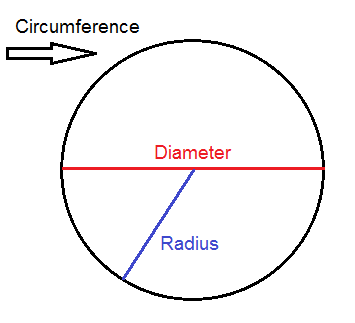# GCSE NON CALC TOPICS

?

## BIDMAS

• Brackets
• Indices (powers)
• Divide
• Multiply
• Subtract
1 of 12

## Fractions

Simplest Form- When you find the smallest number that goes into both the top and bottom,

Example: 6/9 divided by 3 is 2/3.

Adding Fractions- If both the bottom numbers are the same, you just add the top numbers.

Example: 2/3 + 1/3 = 3/3 = 1

If the bottom numbers are different, you need to find a number that both the numbers go into.

Example: 2/3 +1/4. 3 and 4 both go into 12. So you will have 8/12 + 3/12 = 11/12

Subtracting Fractions- Same method as adding fractions.

Example: 2/3 - 1/4. 3 and 4 both go into 12. so you will have 8/12 - 3/12 = 5/12

Multiplying Fractions- Times across. Top x Top. Bottom x Bottom.

Divide Fractions- Flip the Second Number Upsidedown and Times across.

2/3 divided by 1/4. 2/3 x 4/1.    2 x 4 = 8. 3 x1 =3.   =8/3 = 2 and 2/3.

2 of 12

## Area and Circumference of CirclesTo work out the Circumference= Pi x Diameter   or (2xRadius) x Pi.

To work out the Area of Circle = (Pi x Radius) x (Pi x Radius)

3 of 12

## Area of Compound Shapes4 of 12

## Rotation of ShapesYou just need to rotate from centre of rotation.

5 of 12

## Reflection of Shapes.Just flip (mirror image) it over the mirror line given.

6 of 12

## Conversions

1 metre =1,000 millimetres

1\text{ metre} = 100\textbf{ centi}\text{metres}

1,000\text{ metres} = 1\textbf{ kilo}\text{metres}

Centimetres = 10 Millimetres

7 of 12

## Data

• Discrete data (or discrete variables) can only take certain values, e.g. shoe sizes increase in increments of a half: 7, 7.5, 8, 8.5, and so on. You can’t buy size 7.65 shoes.
• Continuous data (or continuous variables) can take any value, e.g. the height of a person can be any number of centimetres: 182cm, 173.4cm, 167.892cm, and so on.
• Primary data is data that you collect first-hand.
• Secondary data is data that was collected in the past by other people.
8 of 12

## Density Mass Volume

Density = Mass Divided by Volume

Mass= Density x Volume

Volume = Density x Mass

9 of 12

## Mean, Mode, Median and Range10 of 12

## Percentages

To find a percentage always divide the original number by 100. then times by the amount.

Example: Find 20% of 150.

150 divided by 100 = 1.5

1.5 x 20 =30.

To make a percentage decimal is simple.

20 % -0.20

35% - 0.35 etc.

To make a percentage a fraction. just put the number over a 100.

20% = 20/100 =2/10 = 1/.

11 of 12

## Ratio Question

Example: Liv and Laura win a lottery of £350,000 and decide to split their winnings according to the ratio 3:4. Work out how much each person receives.

In total, this ratio is made up of

3+4=7 parts. This means that Liv receives \dfrac{3}{7} of the total winnings, and Laura receives \dfrac{4}{7} of the total. So, we get

\text{Liv’s winnings }=\dfrac{3}{7}\times350,000=\pounds150,000

\text{Laura’s winnings }=\dfrac{4}{7}\times350,000=\pounds200,000

12 of 12